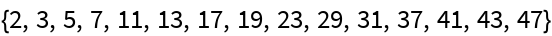#Function Repository Resource:

# SexyPrimes

Get the pairs of primes that differ by 6

Contributed by: Wolfram Staff
 ResourceFunction["SexyPrimes"][n] gives the first n pairs of primes that differ by 6.

## Examples

### Basic Examples

Here are the first 10 pairs of sexy primes:

 In:=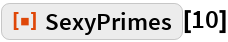Out=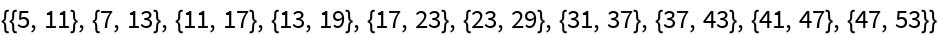Indeed, the differences are 6:

 In:=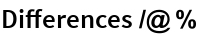Out=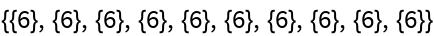For reference, here are the first 20 primes:

 In:=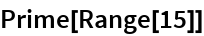Out=